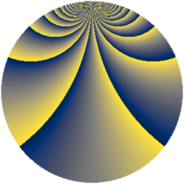# Properties

 Label 3549.2.ckLevel $3549$ Weight $2$ Character orbit 3549.ck Rep. character $\chi_{3549}(272,\cdot)$ Character field $\Q(\zeta_{26})$ Dimension $5760$ Sturm bound $970$

# Related objects

## Defining parameters

 Level: $$N$$ $$=$$ $$3549 = 3 \cdot 7 \cdot 13^{2}$$ Weight: $$k$$ $$=$$ $$2$$ Character orbit: $$[\chi]$$ $$=$$ 3549.ck (of order $$26$$ and degree $$12$$) Character conductor: $$\operatorname{cond}(\chi)$$ $$=$$ $$3549$$ Character field: $$\Q(\zeta_{26})$$ Sturm bound: $$970$$

## Dimensions

The following table gives the dimensions of various subspaces of $$M_{2}(3549, [\chi])$$.

Total New Old
Modular forms 5856 5856 0
Cusp forms 5760 5760 0
Eisenstein series 96 96 0

## Trace form

 $$5760q - 516q^{4} - 26q^{7} - 26q^{9} + O(q^{10})$$ $$5760q - 516q^{4} - 26q^{7} - 26q^{9} - 78q^{15} - 484q^{16} - 26q^{18} - 13q^{21} - 104q^{22} + 412q^{25} - 26q^{28} - 2q^{30} - 14q^{36} - 52q^{37} + 26q^{39} + 31q^{42} - 100q^{43} + 52q^{46} + 2q^{49} + 2q^{51} - 104q^{57} - 26q^{60} + 52q^{63} - 420q^{64} + 208q^{67} - 26q^{72} - 58q^{78} - 124q^{79} + 38q^{81} + 234q^{84} - 52q^{85} - 164q^{88} - 58q^{91} - 26q^{93} + O(q^{100})$$

## Decomposition of $$S_{2}^{\mathrm{new}}(3549, [\chi])$$ into newform subspaces

The newforms in this space have not yet been added to the LMFDB.